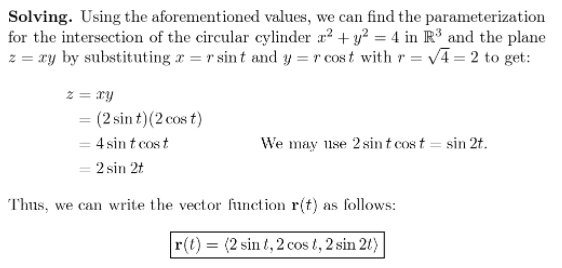# Find A Vector Function, R(T), That Represents The Curve Of Intersection Of The Two Surfaces.

We thoroughly check each answer to a question to provide you with the most correct answers. Found a mistake? Let us know about it through the REPORT button at the bottom of the page.

Find a vector function that represents the curve of intersection of the two surfaces. The cylinder x^2+y^2=4 and the surface z=xy

Answer: r(t) = 2 sin t, 2 cos t, 2 sin 2t.

## Explanation

Rationalization. We know that one way of the parametrization of a circle of radius r in R^2 centered at the origin O (0,0) on the xy-plane is:

x = r sin t  y = r cos t

We can use these values to substitute to the plane z = xy since the circle and the plane are interesting.

Solving.There are many possible answers to this. Another way of parameterizing a circle is by letting x = r cos t and x = r sin t (which is the opposite way but will yield the same z).